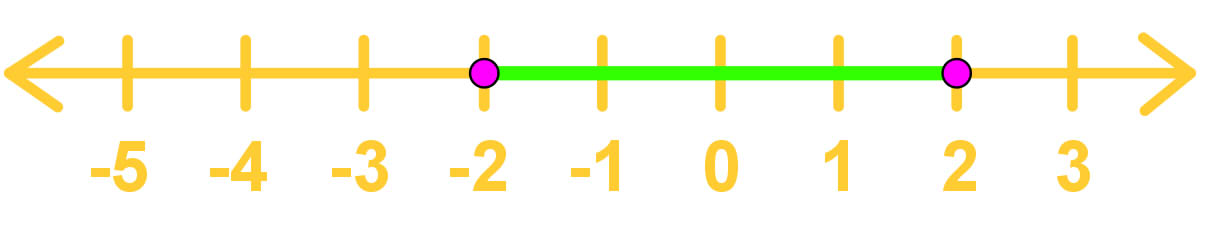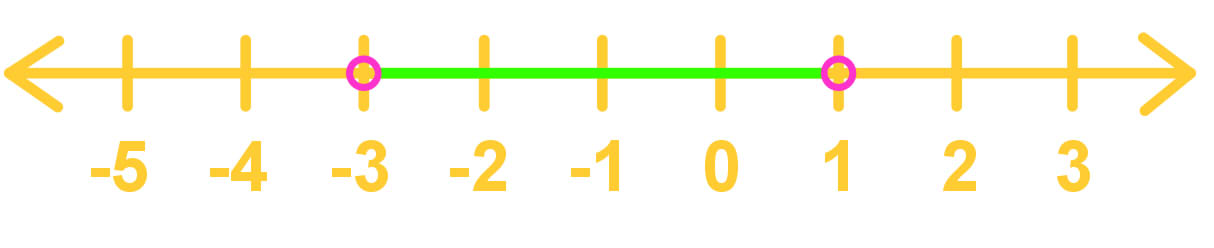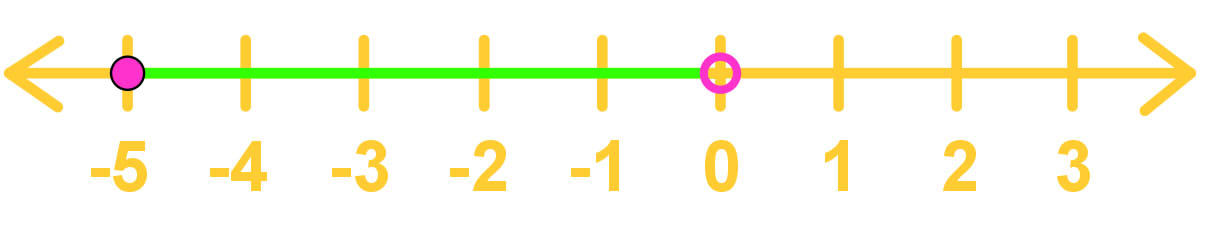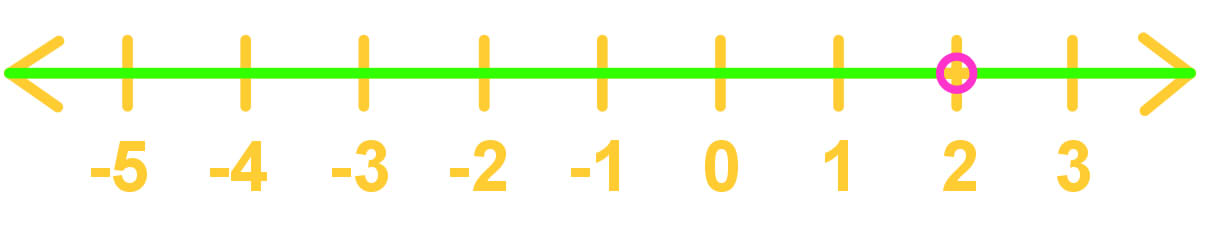# Set builder notation#### Everything You Need in One Place

Homework problems? Exam preparation? Trying to grasp a concept or just brushing up the basics? Our extensive help & practice library have got you covered.#### Learn and Practice With Ease

Our proven video lessons ease you through problems quickly, and you get tonnes of friendly practice on questions that trip students up on tests and finals.#### Instant and Unlimited Help

Our personalized learning platform enables you to instantly find the exact walkthrough to your specific type of question. Activate unlimited help now!

0/1
##### Intros
###### Lessons
1. Introduction to Set Builder Notation

i. What are sets?

ii. Why do we need set builder notations?

0/7
##### Examples
###### Lessons
1. Translating Intervals On Number Lines Into Set Builder Notation Form

Translate the following intervals into set builder notation form.

1.2.3.4.2. Evaluating the Domains of Expressions in Set Builder Notation Form

What are the domains for the following expressions? Write the answers in set builder notation form.

1. $\frac{1}{x}$
2. $\sqrt x$
3. $\frac{2}{x^{2} - 4}$
###### Topic Notes

A set is a collection of elements (usually numbers)

E.g. {$x \in R | x$ > 0} should be read as "the set of all x's that are an element of the real numbers such that x is greater than 0."

Special symbols:

- $R$ = real numbers

- $Z$ = integers

- $N$ = natural numbers

- $Q$ = rational numbers

- $C$ = complex numbers

- $I$ = imaginary numbers